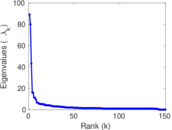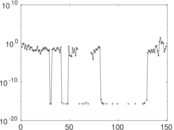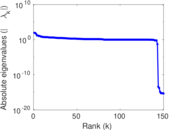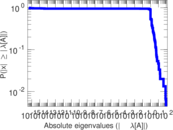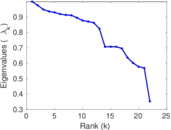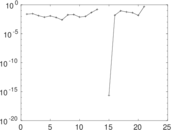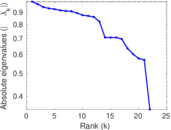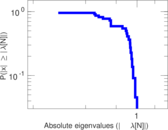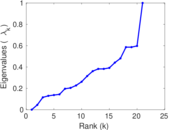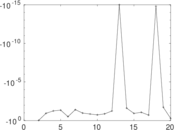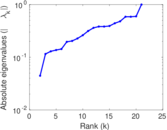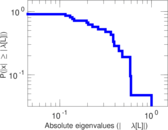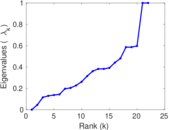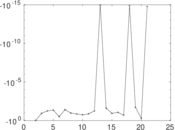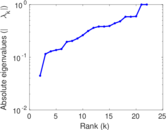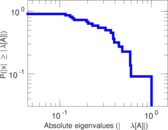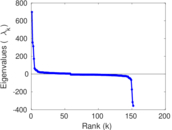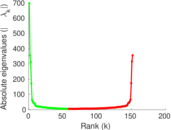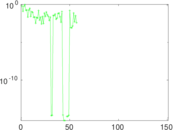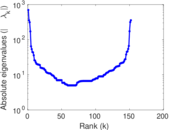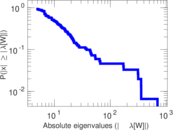# Wikibooks edits (ku)

This is the bipartite edit network of the Kurdish Wikibooks. It contains users and pages from the Kurdish Wikibooks, connected by edit events. Each edge represents an edit. The dataset includes the timestamp of each edit.

 Code `bku` Internal name `edit-kuwikibooks` Name Wikibooks edits (ku) Data source http://dumps.wikimedia.org/ AvailabilityDataset is available for download Consistency checkDataset passed all tests Category Authorship network Dataset timestamp 2017-10-20 Node meaning User, article Edge meaning Edit Network formatBipartite, undirected Edge typeUnweighted, multiple edges Temporal dataEdges are annotated with timestamps

## Statistics

 Size n = 707 Left size n1 = 152 Right size n2 = 555 Volume m = 1,350 Unique edge count m̿ = 655 Wedge count s = 19,263 Claw count z = 1,009,700 Cross count x = 44,383,793 Square count q = 95 4-Tour count T4 = 79,222 Maximum degree dmax = 380 Maximum left degree d1max = 380 Maximum right degree d2max = 122 Average degree d = 3.818 95 Average left degree d1 = 8.881 58 Average right degree d2 = 2.432 43 Fill p = 0.007 764 34 Average edge multiplicity m̃ = 2.061 07 Size of LCC N = 254 Diameter δ = 7 50-Percentile effective diameter δ0.5 = 1.967 47 90-Percentile effective diameter δ0.9 = 3.919 89 Median distance δM = 2 Mean distance δm = 2.970 80 Gini coefficient G = 0.694 587 Balanced inequality ratio P = 0.225 185 Left balanced inequality ratio P1 = 0.165 926 Right balanced inequality ratio P2 = 0.288 148 Relative edge distribution entropy Her = 0.863 529 Power law exponent γ = 5.345 21 Tail power law exponent γt = 2.691 00 Tail power law exponent with p γ3 = 2.691 00 p-value p = 0.018 000 0 Left tail power law exponent with p γ3,1 = 1.941 00 Left p-value p1 = 0.568 000 Right tail power law exponent with p γ3,2 = 3.311 00 Right p-value p2 = 0.003 000 00 Degree assortativity ρ = −0.175 963 Degree assortativity p-value pρ = 5.892 62 × 10−6 Spectral norm α = 89.211 0 Algebraic connectivity a = 0.044 547 6 Spectral separation |λ1[A] / λ2[A]| = 1.116 49 Controllability C = 410 Relative controllability Cr = 0.589 080

## Plots

### Fruchterman–Reingold graph drawing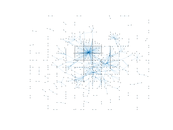### Degree distribution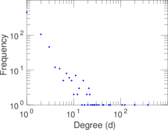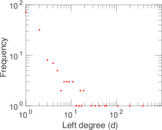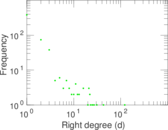### Cumulative degree distribution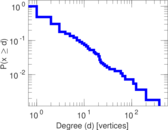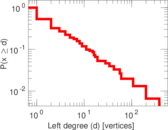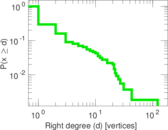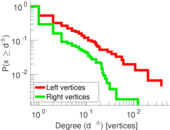### Lorenz curve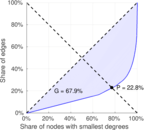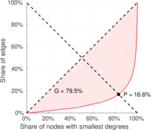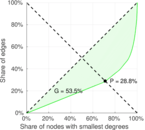### Spectral distribution of the adjacency matrix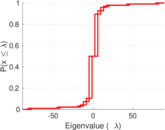### Spectral distribution of the normalized adjacency matrix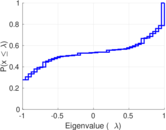### Spectral distribution of the Laplacian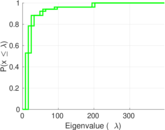### Spectral graph drawing based on the adjacency matrix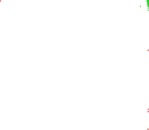### Spectral graph drawing based on the Laplacian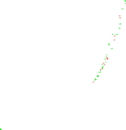### Spectral graph drawing based on the normalized adjacency matrix### Degree assortativity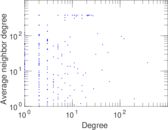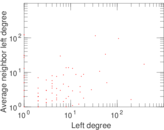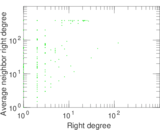### Zipf plot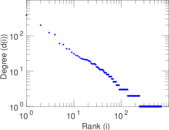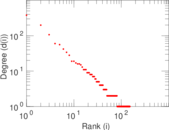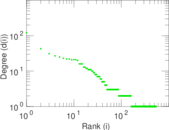### Hop distribution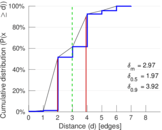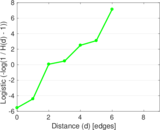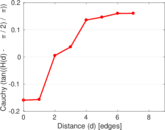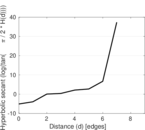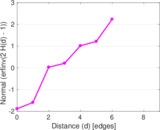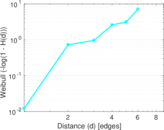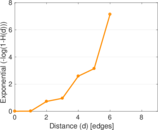### Double Laplacian graph drawing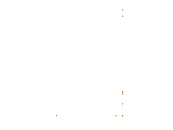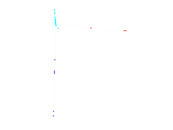### Delaunay graph drawing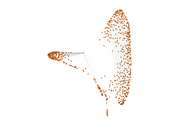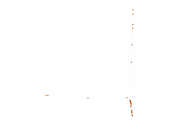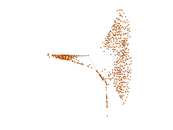### Edge weight/multiplicity distribution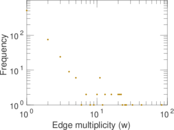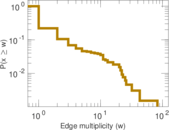### Temporal distribution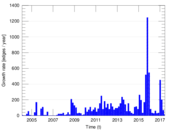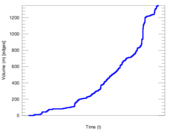### Temporal hop distribution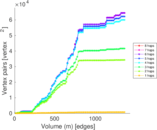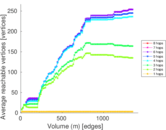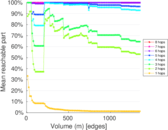### Diameter/density evolution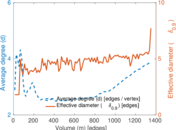### Matrix decompositions plots